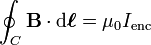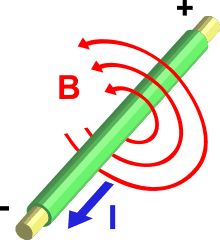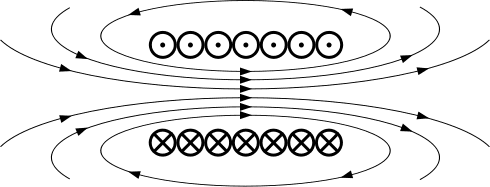# Copper tube and magnet?

• ahhppull
In summary, a magnet moves slowly in a copper tube because of electromagnetic induction, but doesn't in a nonmetal tube. This is due to the existence of eddy currents which produce a magnetic field to oppose the flux change.f

## Homework Statement

Why does a magnet move slowly in a copper tube, but doesn't in a nonmetal tube?

## Homework Equations

Electromagnetic induction (Faraday’s principle) is the production of voltage across a conductor situated in a changing magnetic field or a conductor moving through a stationary magnetic field

Lenz’s Law states that an electromagnetic field interacting with a conductor will generate electrical current that induces a counter magnetic field that opposes the magnetic field generating the current

An eddy current is a swirling current set up in a conductor in response to a changing magnetic field. By Lenz's law, the current swirls in such a way as to create a magnetic field opposing the change; to do this in a conductor, electrons swirl in a plane perpendicular to the magnetic field

## The Attempt at a Solution

I found out that there are two forces repelling each other as the magnet pass the copper tube, but why wouldn't the nonmetal tube create a magnetic force? Is it because nonmetals are bad conductors of electricity? Thanks for the help.

yes. Large current is needed to produce a strong enough magnetic field to slow the falling magnet by a noticeable amount .

In order for eddy currents to exist, you must have mobile charge carriers (The electrons in conducting metal are an example).
The existence of eddy currents produces a magnetic field so as to oppose the magnetic flux change. You get magnetic pressure and the magnet falls much more slowly through the tube.

Yes, according to Amperes law, when there is a current flowing through a conductor, there is also a magnetic field around the conductor.

The formula goes:What this formula means is that the value B is linearly proportional to I , and that's all you need o know for this example if you don't understand integrals.Now, if you round the wire into a coil you gent something like this:where the circles represent a transversal cut through the coil (X represent the current flow away form us, and dots represent current flow towards us). The arrows represent the magnetic field created by the coil.
Now if you put a magnet there it's magnetic field will interact with the coils field, so the magnet will be either pulled into the coil or pushed against.

If a coil is made out of nonmagnetic materials, we presume that they have a endless resistance, which means no current can flow through them. We go back to the formula, B depends linearly on I, if I is 0 so is B. Therefor no magnetic field is made. If you bring the magnet to the coil, it has no other magnetic field to interact with now.

Hope you understood,
feel free to ask if something isn't clear